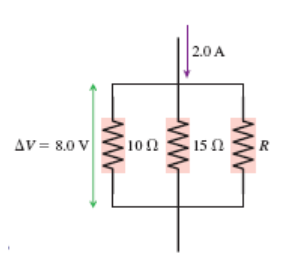# Problem: What is the value of resistor R in the figure below?A) 4.0 ΩB) 12 Ω   C) 36 ΩD) 72 ΩE) 96 Ω

###### FREE Expert Solution

Ohm's law:

$\overline{){\mathbf{V}}{\mathbf{=}}{\mathbf{i}}{\mathbf{R}}}$

Equivalent resistance for parallel resistors:

$\overline{)\frac{\mathbf{1}}{{\mathbf{R}}_{\mathbf{e}\mathbf{q}}}{\mathbf{=}}\frac{\mathbf{1}}{{\mathbf{R}}_{\mathbf{1}}}{\mathbf{+}}\frac{\mathbf{1}}{{\mathbf{R}}_{\mathbf{2}}}{\mathbf{+}}\frac{\mathbf{1}}{{\mathbf{R}}_{\mathbf{3}}}}$

Using ohm's law:

Req = V/i = 8.0/2.0 = 4 Ω###### Problem Details

What is the value of resistor R in the figure below?

A) 4.0 Ω

B) 12 Ω

C) 36 Ω

D) 72 Ω

E) 96 Ω